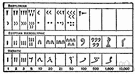numeration system

Also found in: Thesaurus, Encyclopedia.
ThesaurusAntonymsRelated WordsSynonymsLegend:
 Noun 1numeration system - any notation for the representation of numbersmathematical notation - a notation used by mathematicianspositional notation, positional representation system - a numeration system in which a real number is represented by an ordered set of characters where the value of a character depends on its positionradix, base - (numeration system) the positive integer that is equivalent to one in the next higher counting place; "10 is the radix of the decimal system"
References in periodicals archive ?
The Arabic numeration system allows us to discuss and process quantities that are infinitesimally large and small.
The mathematical operations or activities involved could be counting, measuring, drawing, constructions (building) or even more 'complex' ones like the Yoruba numeration system (Zaslavsky, 1994) or finding the length of the hypotenuse of a right angled triangle.
Fan Assessment Numeration System (FANS) design and calibration specifications.
The base ten numeration system that we use is the most
Principles and Standards for School Mathematics (NCTM 2000) states, "It is absolutely essential that students develop a solid understanding of the base-ten numeration system and place-value concepts by the end of grade 2" (p.
Understanding the numeration system and the relation of number to quantity is one of the basic foundations of mathematics.
They designed and built FANS, short for fan assessment numeration system.
understand our numeration system by relating counting, grouping, and place value concepts.
This takes up about half of the book and is a most valuable exercise in its own right, even though, if generally accepted, it may mean a third numeration system superseding Klostermann and Rettberg
b - 1 in our system, it is possible to write any integer N by using the positional numeration system [a.
Solution: The fan assessment numeration system (FANS).
This is one of the important properties of the Base 10 numeration system that seems to be overlooked by students with misconceptions (Steinle & Stacey, 1998).

Site: Follow: Share:
Open / Close